# How to Do Same Calculation for a Same Range in Multiple Sheets in Excel

We often get data exactly in the same type and format from different customers in different Excel worksheets. And we often do the same operation like calculation for these tables. For convenient we can use a simple way to select the same range in multiple sheets in Excel and then do the same operations for the selected ranges, it can avoid we do operations in each worksheet one by one. This article will introduce you how can we select a same range for different sheets and then do the same calculation for them.

In condition, prepare two tables in different worksheet.

Table 1 on sheet1: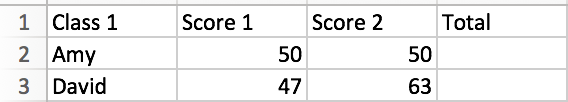Table 2 on sheet2: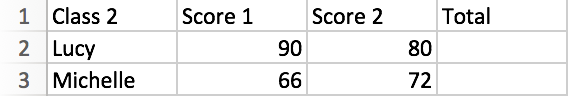We can see that the two tables have the same type and format data, and what we want to do is just calculate the total score for students. In this case we can select the same range and then do sum for them.

Step1: As we want to select the same range (in this case the same range is D2 and D3), we need to select the two sheets together. In MacBook Office-Excel, hold on Command key and then select the two sheets. In other excel versions on Windows OS, you can try to hold on Ctrl key (or Shift key) to select multiple sheets.

In below example, Sheet1 and Shee2 are selected, Sheet3 and Sheet4 are not.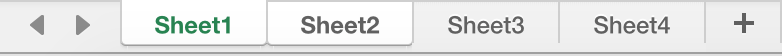Step2: Select D2 and D3 on sheet1 or sheet2, any one is ok. Then you can see on the two sheets, the range is selected.

Table 1 on sheet1: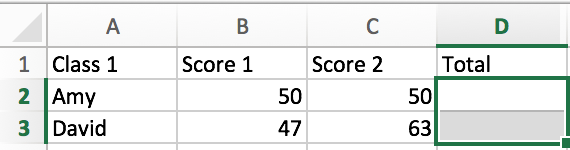Table 2 on sheet2: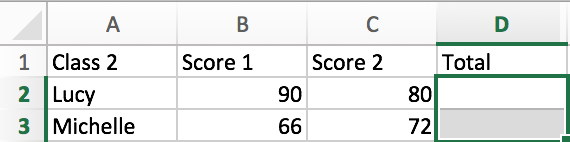Step3: If you want to do the sum for D2 and D3 on both sheets. Just select one sheet, enter the formula in D2, and copy cell to D3, or just drag D2 to D3.

Step4: Switch from the two sheets, you will find that calculations are done for the two tables properly.

Table 1 on sheet1: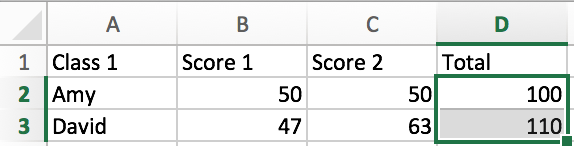Table 2 on sheet2: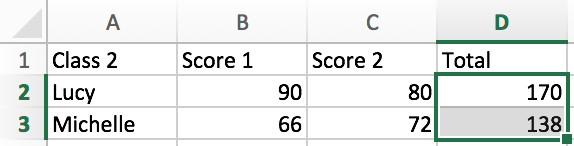Acquire this method can help you a lot in office work for statistic. It can avoid repeat the same operations for the same data on sheets one by one.

Related Posts

Excel Structure Reference

This tutorial introduces the basics of structured referencing in Excel and shows you how to use structured referencing in a table with examples. When you use formulas that reference tables, whether the formula references a part of the table or ...

Excel/Google Sheets: Full Row Reference

Excel or Google sheets supports both full row references and full column references. This article will talk about the usage of full row references. Full Row reference is another cell reference in Excel or Google Sheets, which is used to ...

Excel/Google Sheets: Full Column Reference

Full column reference is another cell reference in Excel or Google Sheets, which is used to reference the entire column, for example, if you want to reference the entire column A, you can use the following reference format A: A. ...

Excel/Google Sheets: Expanding Reference

An expanding reference is a reference type in Excel and Google worksheets that extends the range of a cell when a formula is copied to the cell below or to the right. In this article, we will explain how to ...

Excel/Google Sheets: Mixed Reference

In Microsoft Excel Spreadsheet or Google sheets, there is another cell reference, mixed references, where part of the reference is absolute, part of the relative. This article will describe how to use mixed references through specific examples. Mixed Reference When ...

Sidebar